# Handling Multiple Missing Index Recommendations for the Same Table

Jul 20

## The Problem

Many articles concerning SQL Server missing index recommendations demonstrate the mechanics for obtaining them and often highlight whether the suggested key columns are used in equality or inequality relationships. Most of these examples emphasize a single index that can be implemented to improve performance. However, real-life situations often involve multiple or many suggested indices. For example, during a recent customer study, I observed 28 recommended indices for one table and 52 for another. Clearly, metrics such as improvement measure and user impact frequently can be used to determine the most important recommendations, but sometimes there are either too many similar recommendations – or – improvement measures are almost the same for several proposed indices, which makes determining an optimal index difficult. A large number of recommendations also often results in index proliferation, i.e., missing index recommendations are implemented piecemeal with no overall strategy resulting in tables with 14, 17, or 24 indices as the author observed in a recent customer performance study. The size of the table compounds this problem because it is especially desirable to limit the number of indices on these tables. For example, the table that had 24 indices on it contained over 30 million records.

The following examples of proposed indices that inspired this article illustrate the multiple recommendation phenomenon – RecIndex1: Keys (DateVal), Included Columns (Metric, ReptCat, LocationID, Total_Amount) and RecIndex2: Keys (LocationID, DateVal) Included Columns (Metric, ReptCat, Total_Amount). Clearly, without additional information, it is difficult to determine whether these recommendations must remain separate or could be combined into a single index. In this situation, knowing whether the proposed key columns are used in equality or inequality where clauses can be critical. This article will discuss how to use knowledge of equality and inequality relationships to determine an appropriate course of action.

## Test Table Creation & Load

To determine missing index recommendation behavior, a generic table was constructed and filled with 20 million records. Each record contained an identity column, an ID column, a text column, and 47 metric columns whose values ranged between 1 and 10,000,000. The large number of table columns was used to insure SQL Server would choose an index option when appropriate. Six queries that incorporated various column combinations were executed (some of which differed only in column ordering). To minimize duplication of column values and skewing of query plans, the ABS(cast(CHECKSUM(NewId()) as bigint)) % 10000000 formula was used to generate values that were as random as possible. Two indices were created: a clustered index that used the identity column as its only key and a second nonclustered index that used DupID as its only column. The scripts for the creation, loading, and initial indexing of the table are shown below.

— ##############################################################

— Create test table

— ##############################################################

DROP TABLE FewDuplicates;

CREATE TABLE FewDuplicates (

IDCol bigint identity (20000000,1),

DupID bigint,

MyText varchar(10),

Metric01 bigint, Metric02 bigint, Metric03 bigint, Metric04 bigint,

Metric05 bigint, Metric06 bigint, Metric07 bigint, Metric08 bigint,

Metric09 bigint, Metric10 bigint, Metric11 bigint, Metric12 bigint,

Metric13 bigint, Metric14 bigint, Metric15 bigint, Metric16 bigint,

Metric17 bigint, Metric18 bigint, Metric19 bigint, Metric20 bigint,

Metric21 bigint, Metric22 bigint, Metric23 bigint, Metric24 bigint,

Metric25 bigint, Metric26 bigint, Metric27 bigint, Metric28 bigint,

Metric29 bigint, Metric30 bigint, Metric31 bigint, Metric32 bigint,

Metric33 bigint, Metric34 bigint, Metric35 bigint, Metric36 bigint,

Metric37 bigint, Metric38 bigint, Metric39 bigint, Metric40 bigint,

Metric41 bigint, Metric42 bigint, Metric43 bigint, Metric44 bigint,

Metric45 bigint, Metric46 bigint, Metric47 bigint

)

— ##############################################################

— ##############################################################

declare @DupID bigint = 1

declare @NumRecs bigint = 20000000

truncate table FewDuplicates

set nocount on

while (@DupID <= @NumRecs)

begin

insert into [dbo].[FewDuplicates] (

[DupID], [MyText],

[Metric01], [Metric02], [Metric03], [Metric04], [Metric05], [Metric06], [Metric07], [Metric08], [Metric09], [Metric10], [Metric11], [Metric12], [Metric13], [Metric14], [Metric15], [Metric16], [Metric17], [Metric18], [Metric19], [Metric20], [Metric21], [Metric22], [Metric23], [Metric24], [Metric25], [Metric26], [Metric27], [Metric28], [Metric29], [Metric30], [Metric31], [Metric32], [Metric33], [Metric34], [Metric35], [Metric36], [Metric37], [Metric38], [Metric39], [Metric40], [Metric41], [Metric42],

[Metric43], [Metric44], [Metric45], [Metric46], [Metric47]

)

VALUES (

@DupID,‘my text’,

ABS(cast(CHECKSUM(NewId()) as bigint)) % 10000000,

ABS(cast(CHECKSUM(NewId()) as bigint)) % 10000000,

ABS(cast(CHECKSUM(NewId()) as bigint)) % 10000000,

ABS(cast(CHECKSUM(NewId()) as bigint)) % 10000000,

ABS(cast(CHECKSUM(NewId()) as bigint)) % 10000000,

ABS(cast(CHECKSUM(NewId()) as bigint)) % 10000000,

ABS(cast(CHECKSUM(NewId()) as bigint)) % 10000000,

ABS(cast(CHECKSUM(NewId()) as bigint)) % 10000000,

ABS(cast(CHECKSUM(NewId()) as bigint)) % 10000000,

ABS(cast(CHECKSUM(NewId()) as bigint)) % 10000000,

ABS(cast(CHECKSUM(NewId()) as bigint)) % 10000000,

ABS(cast(CHECKSUM(NewId()) as bigint)) % 10000000,

ABS(cast(CHECKSUM(NewId()) as bigint)) % 10000000,

ABS(cast(CHECKSUM(NewId()) as bigint)) % 10000000,

ABS(cast(CHECKSUM(NewId()) as bigint)) % 10000000,

ABS(cast(CHECKSUM(NewId()) as bigint)) % 10000000,

ABS(cast(CHECKSUM(NewId()) as bigint)) % 10000000,

ABS(cast(CHECKSUM(NewId()) as bigint)) % 10000000,

ABS(cast(CHECKSUM(NewId()) as bigint)) % 10000000,

ABS(cast(CHECKSUM(NewId()) as bigint)) % 10000000,

ABS(cast(CHECKSUM(NewId()) as bigint)) % 10000000,

ABS(cast(CHECKSUM(NewId()) as bigint)) % 10000000,

ABS(cast(CHECKSUM(NewId()) as bigint)) % 10000000,

ABS(cast(CHECKSUM(NewId()) as bigint)) % 10000000,

ABS(cast(CHECKSUM(NewId()) as bigint)) % 10000000,

ABS(cast(CHECKSUM(NewId()) as bigint)) % 10000000,

ABS(cast(CHECKSUM(NewId()) as bigint)) % 10000000,

ABS(cast(CHECKSUM(NewId()) as bigint)) % 10000000,

ABS(cast(CHECKSUM(NewId()) as bigint)) % 10000000,

ABS(cast(CHECKSUM(NewId()) as bigint)) % 10000000,

ABS(cast(CHECKSUM(NewId()) as bigint)) % 10000000,

ABS(cast(CHECKSUM(NewId()) as bigint)) % 10000000,

ABS(cast(CHECKSUM(NewId()) as bigint)) % 10000000,

ABS(cast(CHECKSUM(NewId()) as bigint)) % 10000000,

ABS(cast(CHECKSUM(NewId()) as bigint)) % 10000000,

ABS(cast(CHECKSUM(NewId()) as bigint)) % 10000000,

ABS(cast(CHECKSUM(NewId()) as bigint)) % 10000000,

ABS(cast(CHECKSUM(NewId()) as bigint)) % 10000000,

ABS(cast(CHECKSUM(NewId()) as bigint)) % 10000000,

ABS(cast(CHECKSUM(NewId()) as bigint)) % 10000000,

ABS(cast(CHECKSUM(NewId()) as bigint)) % 10000000,

ABS(cast(CHECKSUM(NewId()) as bigint)) % 10000000,

ABS(cast(CHECKSUM(NewId()) as bigint)) % 10000000,

ABS(cast(CHECKSUM(NewId()) as bigint)) % 10000000,

ABS(cast(CHECKSUM(NewId()) as bigint)) % 10000000,

ABS(cast(CHECKSUM(NewId()) as bigint)) % 10000000,

ABS(cast(CHECKSUM(NewId()) as bigint)) % 10000000

)

set @DupID += 1

end — group option loop

set nocount off

— ##############################################################

— Create indices on the test table

— ##############################################################

CREATE UNIQUE CLUSTERED INDEX [ci_RecID] ON [dbo].[FewDuplicates]

(

[IDCol] ASC

)

WITH (fillfactor = 100, PAD_INDEX = OFF, STATISTICS_NORECOMPUTE = OFF, SORT_IN_TEMPDB = OFF, IGNORE_DUP_KEY = OFF, DROP_EXISTING = OFF, ONLINE = OFF, ALLOW_ROW_LOCKS = ON, ALLOW_PAGE_LOCKS = ON)

ON [PRIMARY]

CREATE NONCLUSTERED INDEX [ix_DupID] ON [dbo].[FewDuplicates]

(

DupID ASC

)

WITH (fillfactor = 100, PAD_INDEX = OFF, STATISTICS_NORECOMPUTE = OFF, SORT_IN_TEMPDB = OFF, IGNORE_DUP_KEY = OFF, DROP_EXISTING = OFF, ONLINE = OFF, ALLOW_ROW_LOCKS = ON, ALLOW_PAGE_LOCKS = ON)

ON [PRIMARY]

## Queries that Create Missing Index Recommendations

To illustrate the issue, two queries were created that were guaranteed to generate missing index recommendations as well as mimic the behavior of the ones cited in The Problem section. They are listed in Table 1 and the differences are highlighted for easier comparison. The query plans for the two queries are displayed in Table 2 and Table 3. Both queries performed full clustered index scans and generated missing index recommendations. The recommendations are shown below in two pieces within each table. The most important points are that Query Plan #1 specifies Metric14 first and Metric43 second, whereas Query Plan #2 specifies Metric43 alone with Metric14 as an included column. At first glance, these appear to be contradictory and potentially incompatible differences.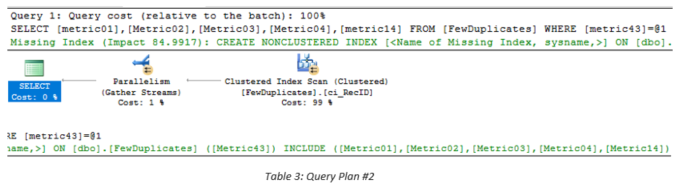Table 4 summarizes the metrics, columns, and full index definitions suggested by SQL Server. Given the very large improvement measure values, the key ordering of the proposed indices, and the somewhat different included columns, it may be tempting to implement both indices despite the fact that the table contained 20 million records. However, more detailed analysis using the data in Table 5 shows that a single index that is constructed properly can accommodate both recommendations and, therefore, both queries. The most important metrics are displayed in column_usage because one uses two equality comparisons and the other only uses one. Therefore, if we specify the equality column used in both queries first and specify the equality/included column second, both queries will be satisfied. VERY IMPORTANT NOTE: Although the key and included column ordering appear obvious because of the column names used in this example table, i.e., suffixes in numerical order, when normal column names like DateVal or LocationID are used, ordering is much less obvious. As cited in my previous blog entitled Query Tuning and Missing Index Recommendations, when ordering is not crucial, e.g., when only equality operations or included columns are specified, SQL Server uses the ordering of the columns in the table itself rather than the ordering specified in the query.

In most cases SQL Server attempts to create covering indices, which are defined to be indices that contain all the columns of a particular query. Please reference the following web page for further information regarding covering indices: https://docs.microsoft.com/en-us/sql/relational-databases/indexes/create-indexes-with-included-columns. In the author’s experience, implementing the keys of a suggested index wtihout the corresponding included columns often results in SQL Server ignoring the new index. Therefore, the included columns are vital to any missing index strategy. Clearly, a point of diminshing returns exists when the number of included columns approaches the total number of columns in the table (especially very large tables), but as long as the number of columns is reasonable, included columns should always be considered. [The queries to obtain the data shown in Table 4 and Table 5 are provided in Table 6.]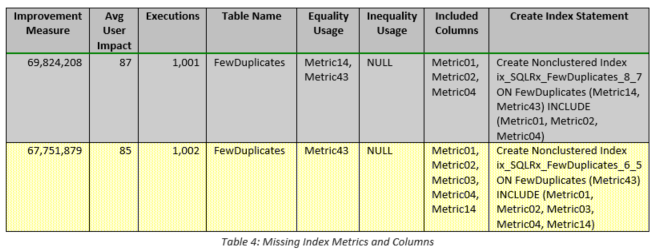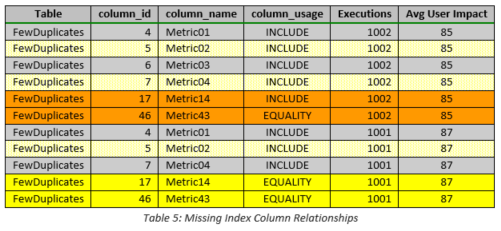SELECT avg_total_user_cost * avg_user_impact * (user_seeks + user_scans)

as [Improvement Measure],

avg_user_impact as [Avg User Impact], user_seeks as Executions,

[statement] as TableName,

equality_columns as [Equality Usage], inequality_columns as [Inequality Usage], included_columns as [Included Columns],

‘Create Nonclustered Index ix_SQLRx_’ + PARSENAME([statement],1) + ‘_’ + CONVERT(varchar, group_handle) + ‘_’ + CONVERT(varchar, g.index_handle) + ‘ ON ‘ +

[statement] +‘ (‘ + ISNULL(replace(equality_columns,‘ ‘,),) +

CASE WHEN equality_columns IS NOT NULL AND inequality_columns IS NOT NULL THEN ‘,’ ELSE END +

ISNULL (replace(inequality_columns,‘ ‘,),) +‘)’ +

CASE WHEN included_columns IS NOT NULL THEN ‘ INCLUDE (‘ + included_columns + ‘)’ ELSE END AS [Create Index Statement]

FROM       sys.dm_db_missing_index_groups g

INNER JOIN   sys.dm_db_missing_index_group_stats s ON

s.group_handle = g.index_group_handle

INNER JOIN   sys.dm_db_missing_index_details d ON d.index_handle = g.index_handle

ORDER BY avg_total_user_cost * avg_user_impact * (user_seeks + user_scans) DESC;

SELECT statement AS [Table], column_id , column_name, column_usage,

migs.user_seeks as Executions, migs.avg_user_impact as [Avg User Impact]

FROM sys.dm_db_missing_index_details AS mid

CROSS APPLY sys.dm_db_missing_index_columns (mid.index_handle)

INNER JOIN sys.dm_db_missing_index_groups AS mig ON mig.index_handle = mid.index_handle

INNER JOIN sys.dm_db_missing_index_group_stats AS migs ON mig.index_group_handle = migs.group_handle

ORDER BY mig.index_group_handle, mig.index_handle, column_id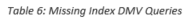Table 7 contains the composite index that satisfies both recommendations and queries. It is important to note that Metric43 is the FIRST key and Metric14 is the second. If this ordering is not followed, Query #1 will perform a full scan. Reviewing both queries demonstrates the following:

1. The key ordering enables the equality operators to apply in both cases.
2. Having Metric14 as the second key satisfies both the equality condition and the included column condition.
3. The other columns specified by the queries are supplied so the table data need never be referenced.

CREATE NONCLUSTERED INDEX ix_CombinedIndex ON [dbo].[FewDuplicates]

(

[Metric43],

[Metric14]

)

INCLUDE (

[Metric01],

[Metric02],

[Metric03],

[Metric04]

)

WITH (fillfactor = 100, PAD_INDEX = OFF, STATISTICS_NORECOMPUTE = OFF, SORT_IN_TEMPDB = OFF, IGNORE_DUP_KEY = OFF, DROP_EXISTING = OFF, ONLINE = OFF, ALLOW_ROW_LOCKS = ON, ALLOW_PAGE_LOCKS = ON)

ON [PRIMARY]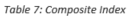Table 8 and Table 9 contain the updated query plans, which illustrate the facts that the clustered index scans have been replaced by index seek operators and the clustered index is not accessed to satisfy either query. Note also that parallelism was present in the query plans shown in Table 2 and Table 3, but is absent in the new query plans displayed in Table 8 and Table 9.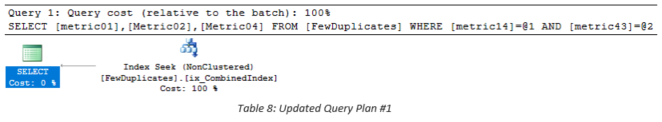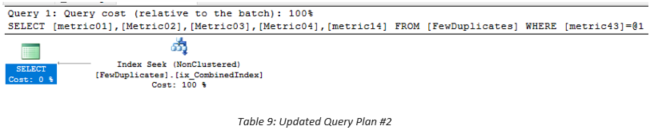## Conclusion

This article illustrated a situation in which multiple missing index recommendations first appeared to necessitate separate indices, resulting in a single index implementation. Careful examination of the key relationships used in the queries and specified by the missing index recommendations enabled the author to develop one composite recommendation that enabled optimal query performance.